# Ohm's law

Ohm's law shows a linear relationship between the voltage and the current in an electrical circuit.

The resistor's voltage drop and resistance set the DC current flow through the resistor.

With water flow analogy we can imagine the electric current as water current through pipe, the resistor as a thin pipe that limits the water flow, the voltage as height difference of the water that enables the water flow.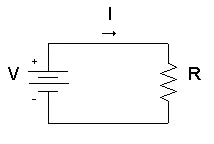## Ohm's law formula

The resistor's current I in amps (A) is equal to the resistor's voltage V in volts (V) divided by the resistance R in ohms (Ω):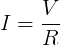V is the voltage drop of the resistor, measured in Volts (V). In some cases Ohm's law uses the letter E to represent voltage. E denotes electromotive force.

I is the electrical current flowing through the resistor, measured in  Amperes (A)

R is the resistance of the resistor, measured in Ohms (Ω)

#### Voltage calculation

When we know the current and resistance, we can calculate the voltage.

The voltage V in volts (V) is equal to the to the current I in amps (A) times the resistance R in ohms (Ω):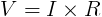#### Resistance calculation

When we know the voltage and the current, we can calculate the resistance.

The resistance R in ohms (Ω) is equal to the voltage V in volts (V) divided by the current I in amps (A):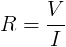Since the current is set by the values of the voltage and resistance, the Ohm's law formula can show that:

• If we increase the voltage, the current will increase.
• If we increase the resistance, the current will reduce.

#### Example #1

Find the current of an electrical circuit that has resistance of 50 Ohms and voltage supply of 5 Volts.

Solution:

V = 5V

R = 50Ω

I = V / R = 5V / 50Ω = 0.1A = 100mA

#### Example #2

Find the resistance of an electrical circuit that has voltage supply of 10 Volts and current of 5mA.

Solution:

V = 10V

I = 5mA = 0.005A

R = V / I = 10V / 0.005A = 2000Ω = 2kΩ

## Ohm's Law for AC Circuit

The load's current I in amps (A) is equal to the load's voltage VZ=V in volts (V) divided by the impedance Z in ohms (Ω):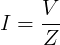V is the voltage drop on the load, measured in Volts (V)

I is the electrical current, measured in Amps (A)

Z is the impedance of the load, measured in Ohms (Ω)

#### Example #3

Find the current of an AC circuit, that has voltage supply of 110V∟70° and load of 0.5kΩ∟20°.

Solution:

V = 110V∟70°

Z = 0.5kΩ∟20° = 500Ω∟20°

I = V / Z = 110V∟70° / 500Ω∟20° = (110V / 500Ω) ∟ (70°-20°) = 0.22A ∟50°

### Ohm's Law Calculator (short form)

Ohm's law calculator: calculates the relation between Voltage, Current and Resistance.

Enter 2 values to get the third value and press the Calculatebutton:

 Enter Resistance: R = ohms (Ω) Enter Current: I = amps (A) Enter Voltage: V = volts (V)

Ohm's law calculator »

Currently, we have around 5610 calculators, conversion tables and usefull online tools and software features for students, teaching and teachers, designers and simply for everyone.